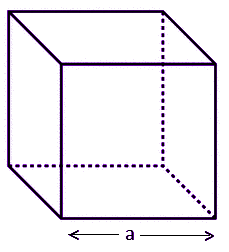Checkout JEE MAINS 2022 Question Paper Analysis : Checkout JEE MAINS 2022 Question Paper Analysis :

# Surface Area Of Cube

Surface area of cube is the sum of areas of all the faces of cube, that covers it. The formula for surface area is equal to six times of square of length of the sides of cube. It is represented by 6a2, where a is the side length of cube.It is basically the total surface area. Also, learn Volume Of A Cube.

When a cube is kept in a three-dimensional space, the area occupied by the sides of the cube in the space is called surface area of cube. In real world, we have been surrounded by many solid objects which have their own area as well volume. The area defines the region occupied by the objects and volume defines the space contained inside that object. The basic solid shapes or 3D shapes which we have learned till now in geometry are:

• Cube
• Cuboid
• Cylinder
• Cone
• Sphere

## Surface Area of Cube Definition

The definition of surface area of a given cube states that if the total surface area is equal to the sum of all the areas of the faces of the cube. Since the cube has six faces, therefore, the total surface area of the cube will be equal to sum of all six faces of cube.

Since, the surface of the cube is in square shape. Hence, area of each face of the cube is equal to square of edge. Let the length of edge of cube is a.

Therefore, area of one face = a2    [By area of square formula]

There are total 6 faces. Therefore,

TSA of cube = a2 + a2 + a2 + a2 + a2 +a2

TSA of cube = 6a2

A cube consists of ‘n’ number of square units. Hence the space covered by these square units on the surface of the cube is the surface area. Basically, the surface area is the sum of all the area of all the shapes that cover the surface of the shape or object. In the case of a cube, there are 6 faces. So the surface area will be sum of all the area of six faces.

Let us derive the formula for surface area for a given cube, to solve problems based on it.

## Surface Area of a Cube Formula

As per the definition of the cube, we know, the cube consists of 6 square faces. Let us consider, a cube whose length of the edges is ‘a’.Now, we know, by the formula of area of a square;

Area = Side= a2

Therefore, the total surface area of a cube = 6 × (area of each side)

= 6 × a2 = 6a2 Square Unit

 TSA = 6 a2

### Length of Edge of the Cube

From the formula of the surface area of the cube, we can also find the length of the edge of the cube by rearranging the formula, such as;

A = 6 (side)2

side2 = A/6

side = √(A/6) = Edge length

where A is the area.

### Examples

Q.1: Calculate the cost required to paint an aquarium which is in cube shape having an edge length of 10m. If the painting cost of an aquarium is INR 3/m2.

Solution: Total surface area of aquarium = 6 (side)2

= 6 (10)2

= 600 sq.m

Total cost of painting the aquarium = 3 × 600 = Rs. 1800

Q.2: If the sidewall of a cubic structure have length 7m, then find the total surface area.

Solution: Given, the length of the sidewall = 7m

As per the formula, we know;

TSA = 6a2

TSA = 6 x 7 x 7 = 294 sq.m

Q.3: Find the length of the edge of the cube, if its area is 2400 sq.cm.

Solution: Given, area = 2400 sq.cm.

We know,

Length of edge of cube = √(A/6) = √(2400/6) = √400 = 20 cm.

To know more about Geometry and surface area of different shapes, you can visit BYJU’S.

## Frequently Asked Questions – FAQs

### How to find the surface area of cube?

Each face of the cube is in square shape. We know the area of square is equal to side2. Since, there are six faces of cube, therefore the total surface area or surface area of a cube will be equal to sum of areas of all its surfaces, i.e.6side2.

### What is the formula of surface area of cube?

If a is the edge length of a surface of a cube, then the formula of surface area is given by 6a2.

### What is the volume of a cube?

The volume of a cube is the space contained by a cube. It is calculated by the formula a3, where a is the edge length of the cube.

### What is the surface area of cube?

The surface area is the region occupied by the surfaces of the cube in a three-dimensional space. The six faces of the cube occupy some space which we need to find using the formula, i.e.6a2, where a is edge length of the cube.

### What is the lateral surface area of cube?

The lateral surface area of cube is the total surface area of the cube, which is equal to sum of areas of all its sides.

Test your knowledge on Surface Area Of Cube

#### 1 Comment

1. Bhavya Shah

It is a good app for clearing the doubts.